Module 20 - Antiderivatives as Indefinite Integrals and Differential Equations

Introduction | Lesson 1 | Lesson 2 | Lesson 3 | Lesson 4 | Self-Test

Lesson 20.1: Antiderivatives as Indefinite Integrals

This lesson explores the relationship between antiderivatives and indefinite integrals and discusses families of curves. You will also investigate finding antiderivatives graphically and analytically.

Defining Indefinite Integrals

Recall that an antiderivative of a function f is a function F whose derivative is f, that is,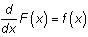.

The Fundamental Theorem of Calculus gives another relationship between an antiderivative F and the function f: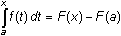where F'(x) = f(x).

If a is a constant, then -F(a) is a constant and we call it C, and the above can be written as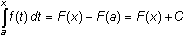.

A modified notation,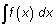, is used to represent all the antiderivatives of f. The new notation is called an indefinite integral. So, the indefinite integral is defined as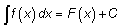where F(x) is any one of the antiderivatives of f(x) and C is an arbitrary constant.Definite vs. Indefinite Integrals A definite integral represents a number when the lower and upper limits are constants. An indefinite integral represents a family of functions all of whose derivatives are equal to f. The difference between any two functions in the family is a constant. There are no limits of integration in an indefinite integral.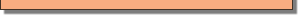Exploring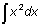Graphically

Suppose you are to find an antiderivative of f(x) = x2. The Fundamental Theorem of Calculus says that by varying the value of a,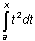will produce a whole family of antiderivatives, so explore the graph of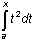for a = 0 and see if you can determine an antiderivative of x2. Letting a = 0 will produce one antiderivative of the family of antiderivatives.

• Enter Y1 = fnInt(T2,T,0,X).
• Graph Y1 in a [-5, 5, 1] x [-5, 5, 1] viewing window.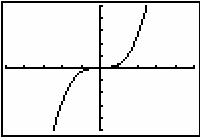This antiderivative looks like f(x) = x3. However, graphing Y2 = X^3 together with the antiderivative in Y1 shows they are not quite the same.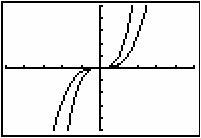The antiderivative in the graph is actually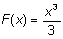. Define Y2 as X^3/3 to provide graphical support thatis an antiderivative of f(x) = x2.

20.1.1 Graph an antiderivative of f(x) = x3 and find an equation for one antiderivative. Support your work graphically.

Checking Indefinite Integrals Analytically

Because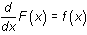you can check an indefinite integral by taking the derivative of the result. For example, the fact that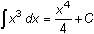can be verified by taking the derivative of the result: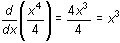.

Because the result of the differentiation is the original function, the integration is confirmed.

The Power Rule for Indefinite Integrals

The generalized version for antidifferentiation where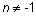is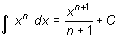, where C is an arbitrary constant.

Because the derivative of a constant is 0, the derivative of the result is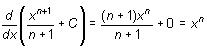Illustrating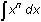The indefinite integral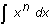may be illustrated by graphing the family of curves that are represented by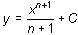for different values of C. For example,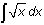corresponds to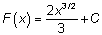. Letting C take the values -240, -200, -160, -120, -80, -40, 0, 40, 80, the family of curves is shown below in a [0, 50,10] x [-50, 100,10] window, where the particular curve associated with C = 0 is the darker curve.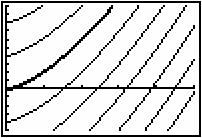Each curve in the family can be obtained by choosing a different value of C and vertically translating the curve corresponding to C = 0.

< Back | Next >